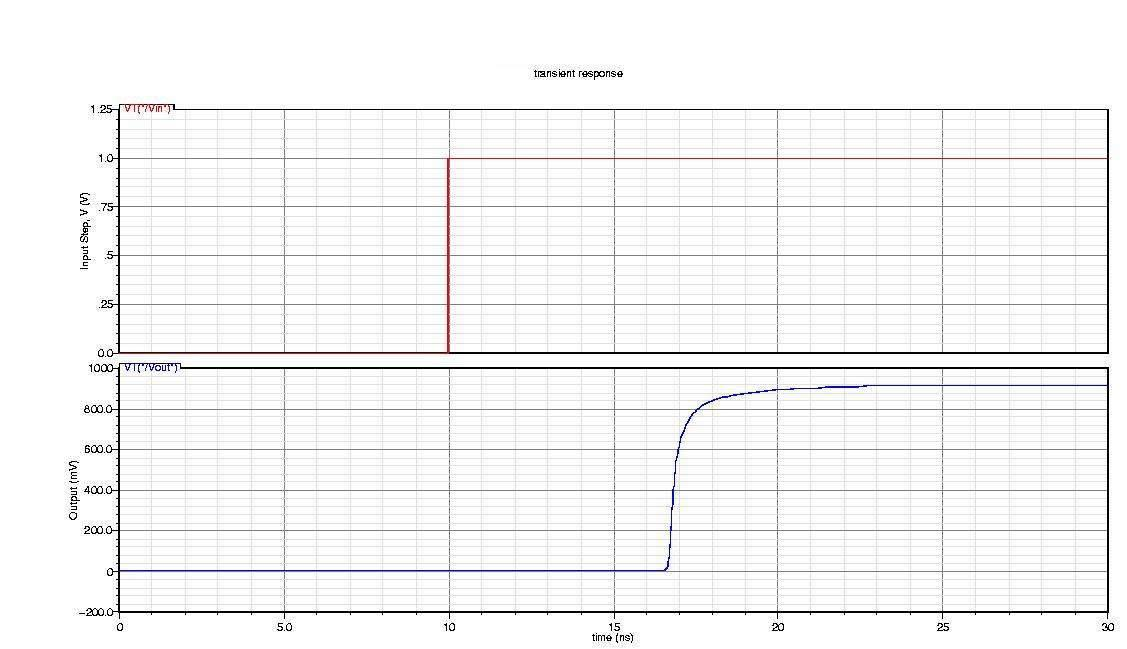Documentation

## Modeling a High-Speed Backplane (Rational Function to a Verilog-A Module)

This example shows how to use RF Toolbox™ functions to generate a Verilog-A module that models the high-level behavior of a high-speed backplane. First, it reads the single-ended 4-port S-parameters for a differential high-speed backplane and converts them to 2-port differential S-parameters. Then, it computes the transfer function of the differential circuit and fits a rational function to the transfer function. Next, the example exports a Verilog-A module that describes the model. Finally, it plots the unit step response of the generated Verilog-A module in a third-party circuit simulation tool.

### Use a Rational Function Object to Describe the High-Level Behavior of a High-Speed Backplane

Read a Touchstone® data file, `default.s4p`, into an `sparameters` object. The parameters in this data file are the 50-ohm S-parameters of a single-ended 4-port passive circuit, measured at 1496 frequencies ranging from 50 MHz to 15 GHz. Then, extract the single-ended 4-port S-parameters from the data stored in the `Parameters` property of the `sparameters` object, use the `s2sdd` function to convert them to differential 2-port S-parameters, and use the `s2tf` function to compute the transfer function of the differential circuit. Then, use the `rationalfit` function to generate an `rfmodel.rational` object that describes the high-level behavior of this high-speed backplane. The `rfmodel.rational` object is a rational function object that expresses the circuit's transfer function in closed form using poles, residues, and other parameters, as described in the `rationalfit` reference page.

```filename = 'default.s4p'; backplane = sparameters(filename); data = backplane.Parameters; freq = backplane.Frequencies; z0 = backplane.Impedance;```

Convert to 2-port differential S-parameters.

```diffdata = s2sdd(data); diffz0 = 2*z0; difftf = s2tf(diffdata,diffz0,diffz0,diffz0);```

Fit the differential transfer function into a rational function.

```fittol = -30; % Rational fitting tolerance in dB delayfactor = 0.9; % Delay factor rationalfunc = rationalfit(freq,difftf,fittol,'DelayFactor',delayfactor)```
```rationalfunc = rfmodel.rational with properties: A: [20x1 double] C: [20x1 double] D: 0 Delay: 6.0172e-09 Name: 'Rational Function' ```

### Export the Rational Function Object as a Verilog-A Module

Use the `writeva` method of the `rfmodel.rational` object to export the rational function object as a Verilog-A module, called `samplepassive1`, that describes the rational model. The input and output nets of `samplepassive1` are called `line_in` and `line_out`. The predefined Verilog-A discipline, `electrical`, describes the attributes of these nets. The format of numeric values, such as the Laplace transform numerator and denominator coefficients, is `%12.10e`. The electrical discipline is defined in the file `disciplines.vams`, which is included in the beginning of the `samplepassive1.va` file.

```workingdir = tempname; mkdir(workingdir) writeva(rationalfunc, fullfile(workingdir,'samplepassive1'), ... 'line_in', 'line_out', 'electrical', '%12.10e', 'disciplines.vams');```
`type(fullfile(workingdir,'samplepassive1.va'));`
```// Module: samplepassive1 // Generated by MATLAB(R) 9.7 and the RF Toolbox 3.7. // Generated on: 26-Aug-2019 12:32:06 `include "disciplines.vams" module samplepassive1(line_in, line_out); electrical line_in, line_out; electrical node1; real nn1[0:1], nn2[0:1], nn3[0:1], nn4[0:1], nn5[0:1], nn6[0:1], nn7[0:1], nn8[0:1], nn9[0:1], nn10[0:0], nn11[0:0]; real dd1[0:2], dd2[0:2], dd3[0:2], dd4[0:2], dd5[0:2], dd6[0:2], dd7[0:2], dd8[0:2], dd9[0:2], dd10[0:1], dd11[0:1]; analog begin @(initial_step) begin nn1 = -3.8392614832e+18; nn1 = 5.2046393014e+07; dd1 = 2.8312609831e+21; dd1 = 3.5124823781e+09; dd1 = 1.0000000000e+00; nn2 = -2.0838483814e+19; nn2 = 5.3487174018e+08; dd2 = 1.8020362314e+21; dd2 = 7.8266367089e+09; dd2 = 1.0000000000e+00; nn3 = 1.7726270794e+19; nn3 = 2.5185716022e+09; dd3 = 1.2157471895e+21; dd3 = 8.1132784895e+09; dd3 = 1.0000000000e+00; nn4 = 2.3112282793e+20; nn4 = 9.2690544437e+08; dd4 = 7.9582429152e+20; dd4 = 1.1379108659e+10; dd4 = 1.0000000000e+00; nn5 = 8.9321469721e+19; nn5 = -1.4945928109e+10; dd5 = 4.1473706594e+20; dd5 = 1.1346735824e+10; dd5 = 1.0000000000e+00; nn6 = -3.5180951909e+20; nn6 = -1.9895507212e+10; dd6 = 1.9080843811e+20; dd6 = 1.0434555792e+10; dd6 = 1.0000000000e+00; nn7 = -1.0593240107e+20; nn7 = 1.9248932577e+10; dd7 = 6.1152960549e+19; dd7 = 1.0001203231e+10; dd7 = 1.0000000000e+00; nn8 = 5.4441539403e+16; nn8 = -9.7818749687e+06; dd8 = 4.3821946493e+19; dd8 = 6.6700188623e+08; dd8 = 1.0000000000e+00; nn9 = 2.2556903052e+16; nn9 = 7.9711163023e+06; dd9 = 2.1228807651e+19; dd9 = 4.9531801417e+08; dd9 = 1.0000000000e+00; nn10 = 1.1592988960e+10; dd10 = 3.0829914556e+09; dd10 = 1.0000000000e+00; nn11 = 1.2852839051e+08; dd11 = 5.9779845807e+08; dd11 = 1.0000000000e+00; end V(node1) <+ laplace_nd(V(line_in), nn1, dd1); V(node1) <+ laplace_nd(V(line_in), nn2, dd2); V(node1) <+ laplace_nd(V(line_in), nn3, dd3); V(node1) <+ laplace_nd(V(line_in), nn4, dd4); V(node1) <+ laplace_nd(V(line_in), nn5, dd5); V(node1) <+ laplace_nd(V(line_in), nn6, dd6); V(node1) <+ laplace_nd(V(line_in), nn7, dd7); V(node1) <+ laplace_nd(V(line_in), nn8, dd8); V(node1) <+ laplace_nd(V(line_in), nn9, dd9); V(node1) <+ laplace_nd(V(line_in), nn10, dd10); V(node1) <+ laplace_nd(V(line_in), nn11, dd11); V(line_out) <+ absdelay(V(node1), 6.0171901584e-09); end endmodule ```

### Plot the Unit Step Response of the Generated Verilog-A Module

Many third-party circuit simulation tools support the Verilog-A standard. These tools simulate standalone components defined by Verilog-A modules and circuits that contain these components. The following figure shows the unit step response of the samplepassive1 module. The figure was generated with a third-party circuit simulation tool.Figure 1: The unit step response.

```delete(fullfile(workingdir,'samplepassive1.va')); rmdir(workingdir)```